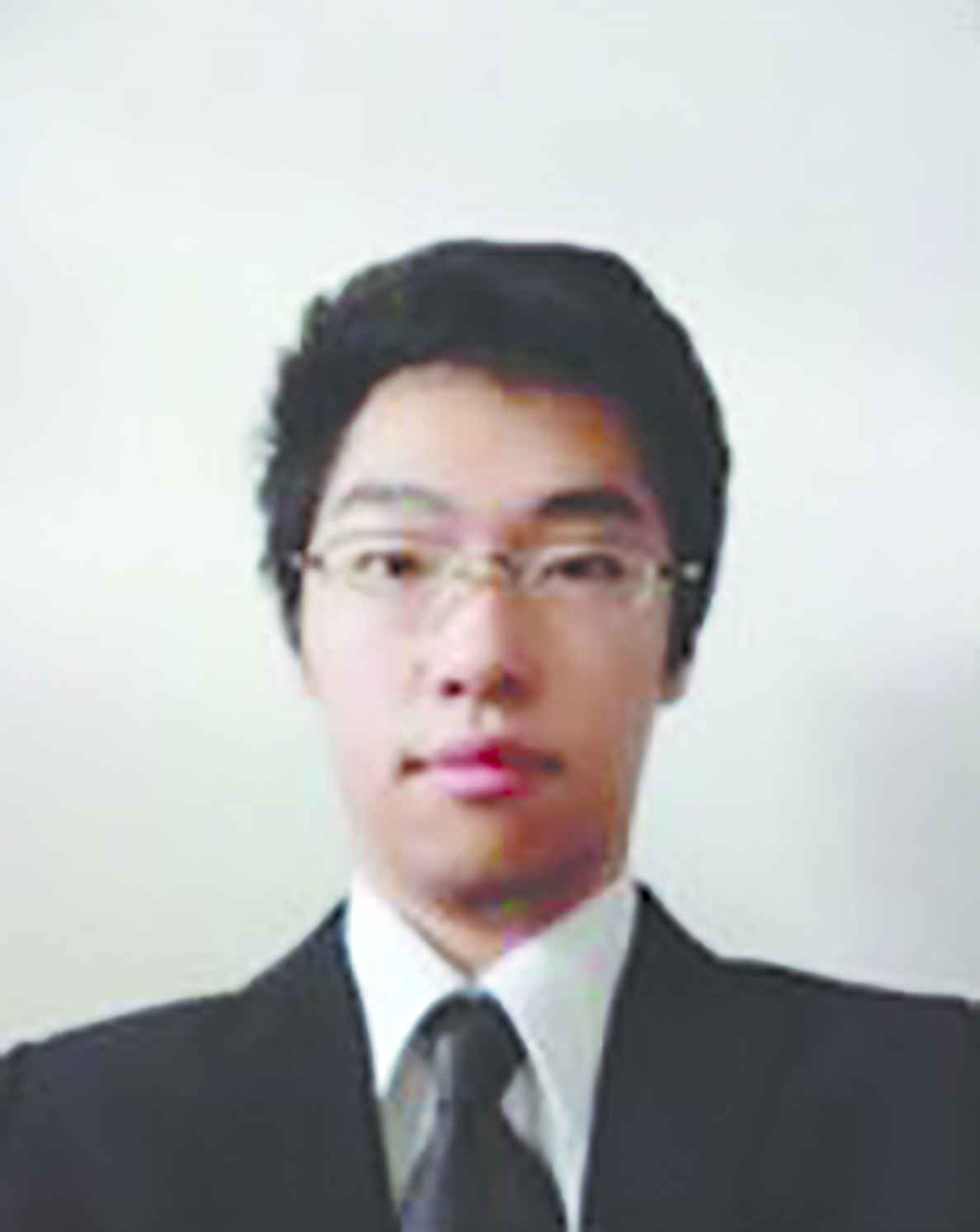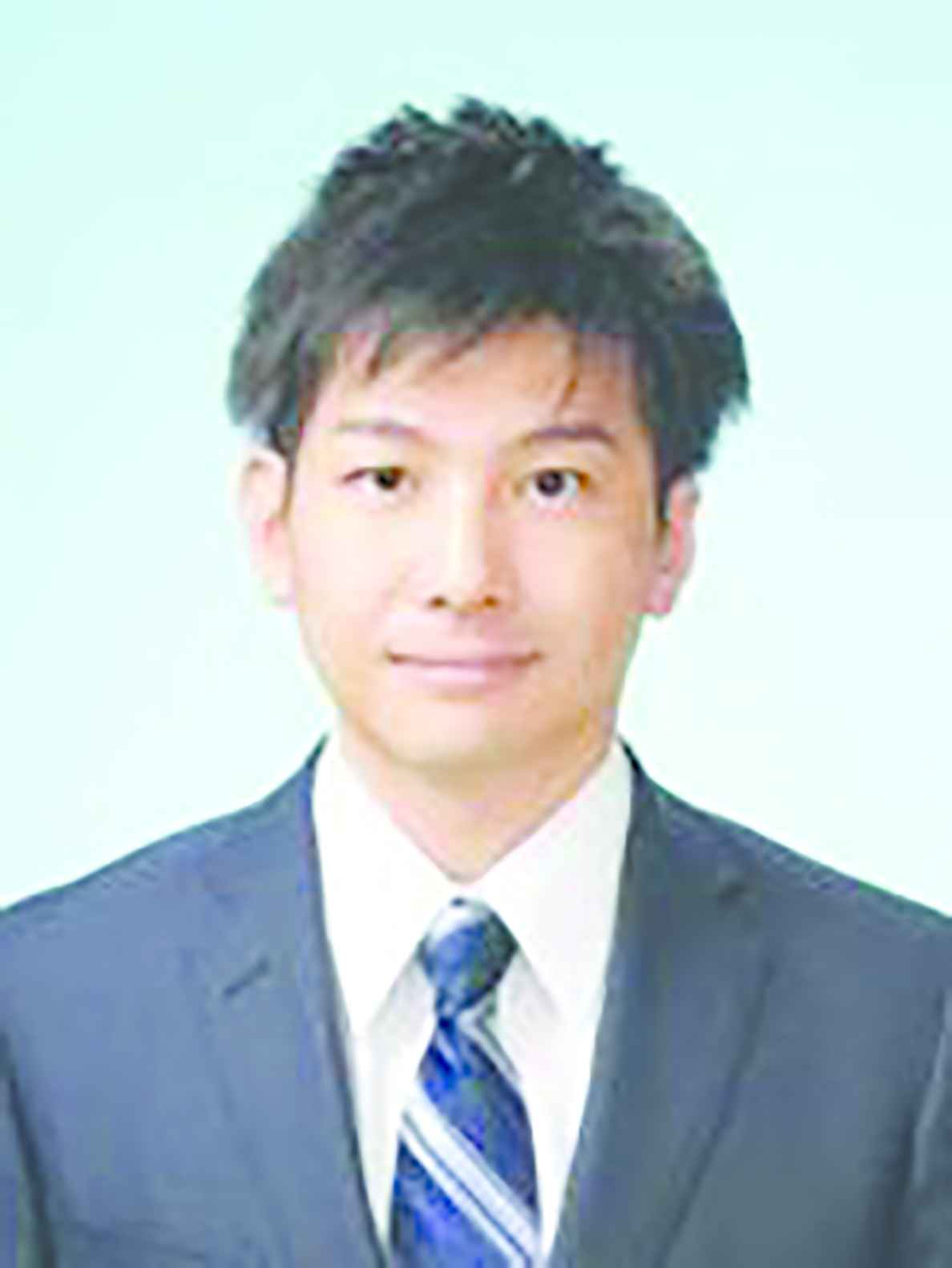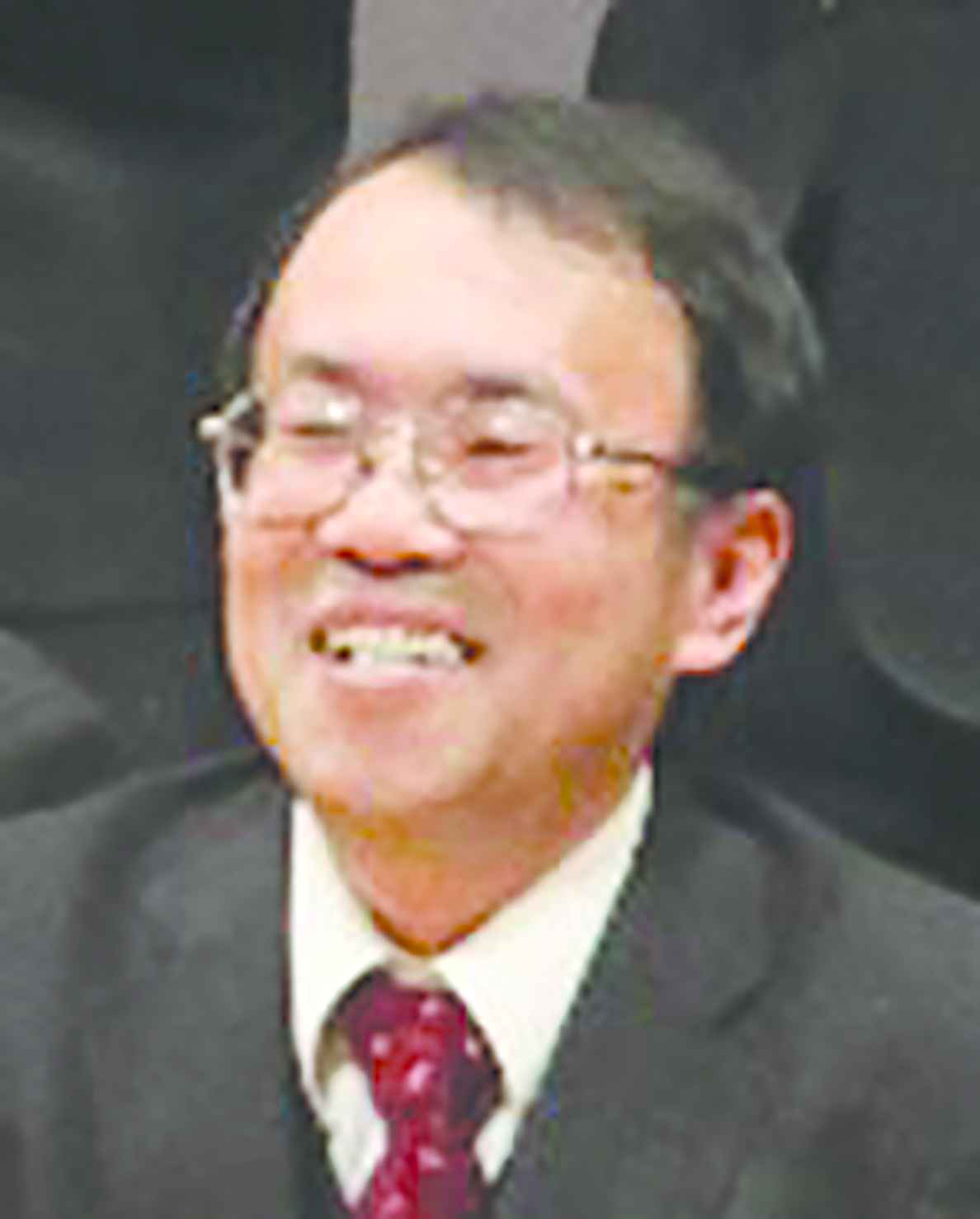# Journal of Robotics, Networking and Artificial Life

Volume 6, Issue 1, June 2019, Pages 33 - 37

# Design of a Performance-Adaptive 1-Parameter Tuning PID Controller

Authors
Yoichiro Ashida1, *, Shin Wakitani2, Toru Yamamoto2
1Department of Electrical Engineering and Computer Science, National Institute of Technology Matsue College, 14-4 Nishiikumacho, Matsue city, Shimane, Japan
2Department of System Cybernetics, Graduate School of Engineering, Hiroshima University, 1-4-1 Kagamiyama, Higashihiroshima city, Hiroshima, Japan
*Corresponding author. Email: yashida@matsue-ct.jp
Corresponding Author
Yoichiro Ashida
Received 16 October 2017, Accepted 10 December 2017, Available Online 25 June 2019.
DOI
10.2991/jrnal.k.190531.007How to use a DOI?
Keywords
PID control; data-oriented; self-tuning control; performance-driven; 1-parameter tuning
Abstract

Many self-tuning Proportional–Integral–Derivative (PID) controllers have been proposed. However, these methods are rarely employed to actual systems because of low reliability of an on-line PID parameter estimator. In this work, a performance adaptive controller that has two stages of PID parameters tuning mechanisms is proposed. One of the tuners is a 1-parameter tuner using the recursive least squares. The other tunes PID parameters using ordinary least squares. The effectiveness of the proposed method is evaluated by an experimental example.

Open Access

## 1. INTRODUCTION

Most of the controllers in industries have fixed control parameters. However, the controllers cannot obtain good control performances against time-variant systems. To tackle this problem, self-tuning controllers [1,2] have been proposed. In these controllers, control parameters are tuned adaptively and can maintain a good control performance to time-variant systems. However, it is difficult to use these methods to actual systems because of low reliability of an on-line estimator. In addition, it is not preferred to tune many control parameters simultaneously in terms of the safeness of a closed-loop system.

Especially in process industries, Proportional–Integral–Derivative (PID) controllers  have been widely used. The reasons why PID controllers have been widely applied include that: (1) PID controllers have simple structure, and (2) physical meanings of the control actions are clear. Therefore, some self-tuning PID control schemes  have been proposed.

In this work, a performance-adaptive controller that has two stages of PID parameters tuning mechanisms is proposed. One of the tuners is a 1-parameter tuner which calculates only the proportional gain using the recursive least squares. The proportional gain depends only on a static gain of the controlled object, thus the reliability of an identification of the proportional gain is higher than the other gains. The other is a PID parameters tuner using ordinary least squares. When the 1-parameter tuner cannot maintain a good control performance, the PID parameters tuner determines new PID parameters to maintain a desired control performance. The effectiveness of the proposed method is evaluated by an experimental example.

## 2. THE TUNING METHOD OF PID PARAMETERS

The PID parameters tuning method is described at this section. A velocity-type PID controller in discrete time domain is given as follows:

Δu(k)=kc{TsTie(k)Δy(k)TdTsΔ2y(k)},
where kc, Ti, and Td are the proportional gain, the integral time and the derivative time, respectively. Δ denotes the differencing operator defined by Δ := 1–z−1, where z−1 is the shift operator which means z−1 y(k) = y(k–1). In addition, u(k) is the input signal and y(k) is the output signal. Furthermore, Ts denotes the sampling time, and e(k) denotes the output error signal defined by e(k) := r(k) – y(k) where r(k) denotes the reference signal. Equation (1) can be rewritten as
Δu(k)=KIe(k)KPΔy(k)KDΔ2y(k),
where KP, KI, and KD are proportional, integral, and derivative gains, respectively. By assuming KI ≠ 0, Equation (3) can be obtained by rewriting Equation (2).
r(k)=1KIΔu(k)+KP+KI+KDKIy(k)KP+2KDKIy(k1)+KDKIy(k2).

Generalized output Φ(k) is defined as follows:

Φ(k)a1Δu(k)+a2{y(k)y(k2)}+a3{y(k1)y(k2)}+y(k2).

Where coefficients a1, a2, and a3 are defined as follows:

a11KIa2KP+KI+KDKIa3KP+2KDKI}.

From (3) to (5), the following relationship can be obtained:

r(k)=Φ(k).

In the proposed method, it is desired to make the system output y(k) track the reference model output yr(k) which is defined by

ym(k)=Gm(z1)r(k),
Gm(z1)z(d+1)P(1)P(z1),
where d denotes the time-delay of the controlled object, and d is assumed to be known. P(z−1) is given as follows:
P(z1)=1+p1z1+p2z2.
Where p1 and p2 are determined by following equations:
p1=2exp(ρ2μ)cos(4μ12μρ)p2=exp(ρμ)ρTsσμ0.25(1δ)+0.51δ}.
σ and δ denote the rise-time and the damping coefficient, respectively, and μ is adjusted by δ. When δ = 0, Gm(z−1) indicates the Binomial model, and when δ = 1, Gm(z−1) indicates the Butterworth model. In practice, the value of δ is considered to design between 0 and 2, and by using bigger δ, the response becomes oscillating.

The evaluation function J is defined as follows:

J=j=0Nε(j)2,
where N denotes the number of data and ε (k) is defined as follows:
ε(k)y(k)Gm(z1)ϕ(k).
By minimizing the evaluation function J, the following relationship can be obtained:
Gm(z1)ϕ(k)y(k).
From Equation (4), parameters to be optimized are ai (i = 1, 2, 3). When the minimization has been finished, it leads the following relationship:
y(k)Gm(z1)ϕ(k),
Therefore, the reference response is realized by using the optimized ai. To apply a PID controller, PID parameters are derived from ai as follows:
kc=2a2+a32a1Ti=(2a2+a32)TsTd=1a2a32a2+a32Ts}.

## 3.1. 1-Parameter Tuning

Both 1-parameter tuning and retuning of all PID parameters are realized by using the PID tuning method described at the previous section. In the 1-parameter tuning, only overall gain of the PID controller kc is tuned. From Equation (15), kc is rewritten as follows:

kc=Tia1Ts.

Furthermore, Ti and Td are constant values, and a2 and a3 are also constant values. Therefore, 1-parameter tuning can be realized by tuning only a1. In this paper, a recursive least squares method  is applied to minimize ε(k). Estimated parameter vector θ^(k) and data vector ψ(k) are defined as follows:

θ^(k)a^1(k),
ψ(k)Gm(z1)Δu(k),
where â1 (k) denotes estimated value of a1 at k steps. The recursive estimation algorithm with forgetting factor  is shown as follows:
θ^(k)=θ^(k1)+K(k)ε(k)
(k)=y(k)Gm(z1)[a2{y(k)y(k2)}+a3{y(k1)y(k2)}+y(k2)],
K(k)=P(k1)ψ(k)ω(k)+ψT(k)P(k1)ψ(k),
P(k)=1ω(k)[P(k1)K(k)ψ(k)TP(k1)].

## 3.2. Control Performance Evaluation

When 1-parameter tuning cannot maintain good control performance, all PID parameters should be tuned. In this subsection, the evaluation criterion of control performance is described. A reference signal is assumed to be the step-type signal. The criterion of control performance is defined as follows:

εGm(k)=1kk0+1i=k0k|Gm(z1)r(i)y(i)|,
where k0 denotes a recent step when a reference signal is changed. εGm(k) denotes an average of errors between controlled output and reference model output. All PID parameters are tuned when εGm(k) satisfies the following condition:
εGm(k)>β|Δr(k0)|,
where α is a user-specified parameter which determines a threshold. |Δr(k0)| is introduced because εGm(k) have proportional relationship to the |Δr(k0)|.

## 3.3. A Method of PID Parameters Tuning

In order to tune all PID parameters, the PID tuning method described at Section 2 are used. The optimized parameters are a1, a2, and a3. In addition, least squares method is used to minimize ε(k). In the ordinary least squares, the data between k0 and k steps are used.

## 4. AN EXPERIMENTAL RESULT

The usefulness of the proposed self-tuning method is experimentally evaluated on a pilot-scale temperature control system. Figure 1 shows an appearance of the controlled object, and the schematic figure is illustrated in Figure 2. In this experimental system, two heaters are secured on a steel plate. These heaters work synchronously, corresponding to the input signal from the computer. One thermo-couple is also prepared, and the measured temperature of the steel is sent to the computer as the system output signal. The control objective is to regulate the temperature of the steel to the desired reference signal by manipulating the power of the heater. In this experimental result, the sampling time Ts is 1 s and the reference signal is changed alternately from 70 (°C) in the temperature and 100 (°C).

To simulate heater deterioration, the relationship between controlled input u(k) and the voltage of the power conditioner v(k) is varied as follows:

v(k)=1+4100u(k)(k200)v(k)=1+43(k200)100600u(k)(200<k800)v(k)=1+1100u(k)(k<800)}.

The control result by using the proposed performance-adaptive controller is shown as Figure 3, and the trajectories of PID parameters corresponding to Figure 3 is shown in Figure 4. In this result, the initial PID parameters were determined by using the PID tuning method described at Section 2 as follows:

kc(0)=0.70,Ti(0)=28.9,Td(0)=4.67.

Parameters of the proposed method were set as follows: α = 1, β = 0.2, d = 8, w(k) = 0.995, σ = 30, and δ = 0. From Equation (26), initial estimated parameter was determined as θ^(0)=41.3 .

Figure 3 indicates that the control performance gradually deteriorated after 200 steps. Figure 4 shows that only kc was tuned before 863 steps, and all PID parameters are tuned at 863 steps. The proportional gain kc became larger on the 1-parameter tuning period. Considering that the system gain became smaller on the period as Equation (25), the variation of the kc is correct. In addition, after the tuning of the all parameters, the trajectory of the control output is likely the same as the reference trajectory.

## 5. CONCLUSION

In this paper, a performance-adaptive 1-parameter tuning PID controller is proposed, and effectiveness of the proposed method is evaluated by the pilot-scale temperature control system. The proposed performance adaptive controller has two stages of PID parameters tuning mechanisms. The features of the proposed method are summarized as follows:

• The proposed method is the 1-parameter tuning method, and only kc is tuned.

• All PID parameters are retuned only when control performance deteriorates.

• Both the 1-parameter tuning and the retuning method of all PID parameters are based on the same PID tuning scheme.

From these characteristics, the proposed method can be easily understood. However, the effectiveness of the 1-parameter tuning in this paper does not be proved analytically. This is a future work.

## CONFLICTS OF INTEREST

There is no conflicts of interest.

## Authors Introduction

Dr. Yoichiro AshidaHe received his D.Eng. degree from Hiroshima University in 2019. His main research interests are data-driven controller tuning, PID control, and delay systems. He is currently an Assistant Professor with Department of Electrical Engineering and Computer Science, National Institute of Technology Matsue College.

Dr. Shin WakitaniHe received his B.Ed. and M.Ed. degrees from Hiroshima University in 2009 and 2011, respectively, and his D.Eng. degree from Hiroshima University in 2013. From 2013 to 2016, he was with Tokyo University of Agriculture and Technology as an Assistant Professor. He is currently an Associate Professor with Department of System Cybernetics, Graduate School of Engineering, Hiroshima University. His research interests are in data-driven controller design, performance-driven controller design, and controller design based on skilled workers’ operation data.

Dr. Toru YamamotoHe received his B.Eng. and M.Eng. degrees from the University of Tokushima, Japan, in 1984 and 1987, respectively, and the D.Eng. degree from Osaka University, Japan, in 1994. He is currently a Professor with the Department of System Cybernetics, Graduate School of Engineering, Hiroshima University, Japan. He was a Visiting Researcher with the Department of Mathematical Engineering and Information Physics, University of Tokyo, Japan, in 1991, and he was an Overseas Research Fellow of the Japan Society for Promotion of Science (JSPS) with the University of Alberta for six months in 2006. His current research interests are in the area of self-tuning and learning control, data-driven control, and their implementation for industrial systems. He was a General Chair of ADCONIP 2014 and SICE Annual Conference 2019.